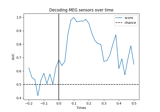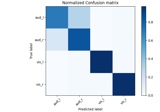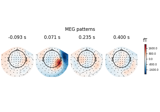# mne.decoding.Vectorizer#

class mne.decoding.Vectorizer[source]#

Transform n-dimensional array into 2D array of n_samples by n_features.

This class reshapes an n-dimensional array into an n_samples * n_features array, usable by the estimators and transformers of scikit-learn.

Examples

clf = make_pipeline(SpatialFilter(), _XdawnTransformer(), Vectorizer(),

LogisticRegression())

Attributes:
features_shape_`tuple`

Stores the original shape of data.

Methods

 `fit`(X[, y]) Store the shape of the features of X. `fit_transform`(X[, y]) Fit the data, then transform in one step. Transform 2D data back to its original feature shape. Convert given array into two dimensions.
fit(X, y=None)[source]#

Store the shape of the features of X.

Parameters:
Xarray_like

The data to fit. Can be, for example a list, or an array of at least 2d. The first dimension must be of length n_samples, where samples are the independent samples used by the estimator (e.g. n_epochs for epoched data).

y`None` | `array`, shape (n_samples,)

Used for scikit-learn compatibility.

Returns:
selfinstance of `Vectorizer`

Return the modified instance.

fit_transform(X, y=None)[source]#

Fit the data, then transform in one step.

Parameters:
Xarray_like

The data to fit. Can be, for example a list, or an array of at least 2d. The first dimension must be of length n_samples, where samples are the independent samples used by the estimator (e.g. n_epochs for epoched data).

y`None` | `array`, shape (n_samples,)

Used for scikit-learn compatibility.

Returns:
X`array`, shape (n_samples, -1)

The transformed data.

inverse_transform(X)[source]#

Transform 2D data back to its original feature shape.

Parameters:
Xarray_like, shape (n_samples, n_features)

Data to be transformed back to original shape.

Returns:
X`array`

The data transformed into shape as used in fit. The first dimension is of length n_samples.

transform(X)[source]#

Convert given array into two dimensions.

Parameters:
Xarray_like

The data to fit. Can be, for example a list, or an array of at least 2d. The first dimension must be of length n_samples, where samples are the independent samples used by the estimator (e.g. n_epochs for epoched data).

Returns:
X`array`, shape (n_samples, n_features)

The transformed data.

## Examples using `mne.decoding.Vectorizer`#Decoding (MVPA)

Decoding (MVPA)XDAWN Decoding From EEG data

XDAWN Decoding From EEG dataLinear classifier on sensor data with plot patterns and filters

Linear classifier on sensor data with plot patterns and filters